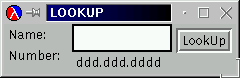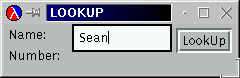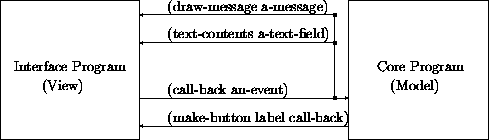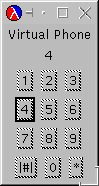# Section 22Designing Abstractions with First-Class Functions

We have seen that functions can consume functions and how important that is for creating single points of control in a function. But functions not only can consume functions, they can also produce them. More precisely, expressions in the new Scheme can evaluate to functions. Because the body of a function definition is also an expression, a function can produce a function. In this section, we first discuss this surprising idea and then show how it is useful for abstracting functions and in other contexts.

## 22.1  Functions that Produce Functions

While the idea of producing a function may seem strange at first, it is extremely useful. Before we can discuss the usefulness of the idea, though, we must explore how a function can produce a function. Here are three examples:

```(define (f x) first)
(define (g x) f)
(define (h x)
(cond
((empty? x) f)
((cons? x) g)))
```

The body of `f` is `first`, a primitive operation, so applying `f` to any argument always evaluates to `first`. Similarly, the body of `g` is `f`, so applying `g` to any argument always evaluates to `f`. Finally, depending on what kind of list we supply as an argument to `h`, it produces `f` or `g`.

None of these examples is useful but each illustrates the basic idea. In the first two cases, the body of the function definition is a function. In the last case, it evaluates to a function. The examples are useless because the results do not contain or refer to the argument. For a function `f` to produce a function that contains one of `f`'s arguments, `f` must define a function and return it as the result. That is, `f`'s body must be a local-expression.

Recall that local-expressions group definitions and ask DrScheme to evaluate a single expression in the context of these definitions. They can occur wherever an expression can occur, which means the following definition is legal:

```(define (add x)
(local ((define (x-adder y) (+ x y)))
```

The function `add` consumes a number; after all, `x` is added to `y`. It then defines the function `x-adder` with a local-expression. The body of the local-expression is `x-adder`, which means the result of `add` is `x-adder`.

To understand `add` better, let us look at how an application of `add` to some number evaluates:

```  (define f (add 5))
= (define f (local ((define (x-adder y) (+ 5 y)))
= (define f (local ((define (x-adder5 y) (+ 5 y)))
= (define (x-adder5 y) (+ 5 y))
```

The last step adds the function `x-adder5` to the collection of our definitions; the evaluation continues with the body of the local-expression, `x-adder5`, which is the name of a function and thus a value. Now `f` is defined and we can use it:

```  (f 10)
= (+ 5 10)
= 15
```

That is, `f` stands for `x-adder5`, a function, which adds `5` to its argument.

Using this example, we can write `add`'s contract and a purpose statement:

```;; `add : number  ->  (number  ->  number)`
;; to create a function that adds `x` to its input
(local ((define (x-adder y) (+ x y)))
```

The most interesting property of `add` is that its result ``remembers'' the value of `x`. For example, every time we use `f`, it uses `5`, the value of `x`, when `add` was used to define `f`. This form of ``memory'' is the key to our simple recipe for defining abstract functions, which we discuss in the next section.

## 22.2  Designing Abstractions with Functions-as-Values

The combination of local-expressions and functions-as-values simplifies our recipe for creating abstract functions. Consider our very first example in figure 53 again. If we replace the contents of the boxes with `rel-op`, we get a function that has a free variable. To avoid this, we can either add `rel-op` to the parameters or we can wrap the definition in a `local` and prefix it with a function that consumes `rel-op`. Figure 59 shows what happnes when we use this idea with `filter`. If we also make the `local`ly defined function the result of the function, we have defined an abstraction of the two original functions.

 ```(define (filter2 rel-op) (local ((define (abs-fun alon t) (cond [(empty? alon) empty] [else (cond [(rel-op (first alon) t) (cons (first alon) (abs-fun (rest alon) t))] [else (abs-fun (rest alon) t)])]))) abs-fun)) ``` Figure 59:  Abstracting with local

Put differently, we follow the example of `add` in the preceding section. Like `add`, `filter2` consumes an argument, defines a function, and returns this function as a result. The result remembers the `rel-op` argument for good as the following evaluation shows:

```  (filter2 <)
```

```= (local ((define (abs-fun alon t)
(cond
[(empty? alon) empty]
[else
(cond
[(< (first alon) t)
(cons (first alon)
(abs-fun (rest alon) t))]
[else
(abs-fun (rest alon) t)])])))
abs-fun)
```

```= (define (below3 alon t)
(cond
[(empty? alon) empty]
[else
(cond
[(< (first alon) t)
(cons (first alon)
(below3 (rest alon) t))]
[else
(below3 (rest alon) t)])]))
below3
```

Remember that as we lift a `local` definition to the top-level definitions, we also rename the function in case the same `local` is evaluated again. Here we choose the name `below3` to indicate what the function does. And indeed, a comparison between `below` and `below3` reveals that the only difference is the name of the function.

From the calculation, it follows that we can give the result of `(filter2 <)` a name and use it as if it were `below`. More succinctly,

```(define below2 (filter2 <))
```

is equivalent to

```(define (below3 alon t)
(cond
[(empty? alon) empty]
[else
(cond
[(< (first alon) t)
(cons (first alon)
(below3 (rest alon) t))]
[else
(below3 (rest alon) t)])]))

(define below2 below3)
```

which means `below2` is just another name for `below3` and which directly proves that our abstract function correctly implements `below`.

The example suggests a variant of the abstraction recipe from section 21:

The comparison:
The new recipe still requires us to compare and to mark the differences.

The abstraction:
The new step concerns the way we define the abstract function. We place one of the functions into a local-expression and use the name of the function as the body of the `local`:
```(local ((define (concrete-fun x y z)
... op1 ... op2 ...))
concrete-fun)
```

From that, we can create the abstract function by listing the names in the boxes as parameters:

```(define (abs-fun op1 op2)
(local ((define (concrete-fun x y z)
... op1 ... op2 ...))
concrete-fun))
```

If `op1` or `op2` is a special symbol, say `<`, we name it something that is more meaningful in the new context.

The test:
To test the abstract function, we define the concrete functions again, as before. Consider the example of `below` and `above`. Obtaining `below` and `above` as instances of `filter2` is now straightforward:
```(define below2 (filter2 <))

(define above2 (filter2 >))
```

We simply apply `filter2` to the contents of the box in the respective concrete function and that application produces the old function.

The contract:
The contract of an abstract function contains two arrows. After all, the function produces a function, and to describe this relationship the type to the right of the first arrow must contain another arrow.

Here is the contract for `filter2`:

```;; `filter2 : (X Y  ->  boolean)  ->  ((listof X) Y  ->  (listof X))`
```

It consumes a comparison function and produces a concrete filter-style function.

The generalization of the contract works as before.

Given our experience with the first design recipe, the second one is only a question of practice.

Exercise 22.2.1.   Define an abstraction of the functions `convertCF` and `names` from section 21.1 using the new recipe for abstraction.Solution

Exercise 22.2.2.   Define an abstract version of `sort` (see exercise 19.1.6) using the new recipe for abstraction.Solution

Exercise 22.2.3.   Define `fold` using the new recipe for abstraction. Recall that `fold` abstracts the following pair of functions:

 ```;; `sum : (listof number)  ->  number` ;; to compute the sum of `alon` (define (sum alon) (cond [(empty? alon) 0] [else (+ (first alon) (sum (rest alon)))])) ``` ```;; `product : (listof number)  ->  number` ;; to compute the product of `alon` (define (product alon) (cond [(empty? alon) 1] [else (* (first alon) (product (rest alon)))])) ```Solution

## 22.3  A First Look at Graphical User Interfaces

Functions as first-class values play a central role in the design of graphical user interfaces. The term ``interface'' refers to the boundary between the program and a user. As long as we are the only users, we can apply functions to data in DrScheme's Interactions window. If we want others to use our programs, though, we must provide a way to interact with the program that does not require any programming knowledge. The interaction between a program and a casual user is the USER INTERFACE.

A GRAPHICAL USER INTERFACE (GUI) is the most convenient interface for casual users. A GUI is a window that contains GUI items. Some of these items permit users to enter text; others are included so that users can apply a specific function; and yet others exist to display a function's results. Examples include buttons, which the user can click with the mouse and which trigger a function application; choice menus, from which the user can choose one of a collection of values; text fields, into which the user can type arbitrary text; and message fields, into which a program can draw text.Figure 60:  A simple GUI for looking up a phone number

Take a look at the simple GUI in figure 60. The left-most picture shows its initial state. In that state, the GUI contains a text field labeled ``Name'' and a message field labeled ``Number'' plus a ``LookUp'' button. In the second picture, the user has entered the name ``Sean'' but hasn't yet clicked the ``LookUp'' button.48 Finally, the right-most picture shows how the GUI displays the phone number of ``Sean'' after the user clicks the ``LookUp'' button.

The core of the program is a function that looks up a phone number for a name in a list. We wrote several versions of this function in part II but always used it with DrScheme's Interactions window. Using the GUI of figure 60, people who know nothing about Scheme can now use our function, too.

To build a graphical user interface, we build structures49 that correspond to the GUI items and hand them over to a GUI manager. The latter constructs the visible window from these items. Some of the structures' fields describe the visual properties of the GUI's elements, such as the label of a button, the initial content of a message field, or the available choices on a menu. Other fields stand for functions. They are called CALL-BACK FUNCTIONS because the GUI manager calls -- or applies -- these functions when the user manipulates the corresponding GUI element. Upon application, a call-back function obtains strings and (natural) numbers from the elements of the GUI and then applies the function proper. This last step computes answers, which the call-back function can place into GUI elements just like graphics functions draw shapes on a canvas.Figure 61:  The model-view arrangement

The ideal program consists of two completely separate components: the MODEL, which is the kind of program we are learning to design, and a VIEW, which is the GUI program that manages the display of information and the user's mouse and keyboard manipulations. The bridge between the two is the CONTROL expression. Figure 61 graphically illustrates the organization, known as the MODEL-VIEW-CONTROL architecture. The lowest arrow indicates how a program makes up a button along with a call-back function. The left-to-right arrow depicts the mouse-click event and how it triggers an application of the call-back function. It, in turn, uses other GUI functions to obtain user input before it applies a core function or to display results of the core function.

The separation of the program into two parts means that the definitions for the model contain no references to the view, and that the definitions for the view contain no references to the data or the functionality of the model. The organization principle evolved over two decades from many good and bad experiences. It has the advantage that, with an adaptation of just the bridge expression, we can use one and the same program with different GUIs and vice versa. Furthermore, the construction of views requires different tools than does the construction of models. Constructing views is a labor-intensive effort and involves graphical design, but fortunately, it is often possible to generate large portions automatically. The construction of models, in contrast, will always demand a serious program design effort.

 A gui-item is either `(make-button string (X  ->  true))` `(make-text string)` `(make-choices (listof string))` or `(make-message string)`. ```;; `create-window : (listof (listof gui-item))  ->  true` ;; to add gui-items to the window and to show the window ;; each list of gui-items defines one row of gui items in the window ;; `hide-window : X  ->  true` ;; to hide the window ;; `make-button : string (event%  ->  true)  ->  gui-item` ;; to create a button with label and call-back function ;; `make-message : string  ->  gui-item` ;; to create an item that displays a message ;; `draw-message : gui-item[message%] string  ->  true` ;; to display a message in a message item ;; it erases the current message ;; `make-text : string  ->  gui-item` ;; to create an item (with label) that allows users to enter text ;; `text-contents : gui-item[text%]  ->  string` ;; to determine the contents of a text field ;; `make-choice : (listof string)  ->  gui-item` ;; to create a choice menu that permits users to choose from some ;; string alternatives ;; `choice-inded : gui-item[choice%]  ->  num` ;; to determine which choice is currently selected in a choice-item ;; the result is the 0-based index in the choice menu ``` Figure 62:  The gui.ss operations

Here we study the simplified GUI world of the teachpack gui.ss. Figure 62 specifies the operations that the teachpack provides.50 The GUI manager is represented by the function `create-window`. Its contract and purpose statement are instructive. They explain that we create a window from a list. The function arranges these lists in a corresponding number of rows on the visible window. Each row is specified as a list of `gui-item`s. The data definition for `gui-item`s in figure 62 shows that there are four kinds:

text fields,
which are created with `(make-text a-string)` and allow users to enter arbitrary text into an area in the window;

buttons,
which are created with ```(make-button a-string a-function)``` and allow users to apply a function with the click of a mouse button;

which are created with ```(make-choice a-list-of-strings)``` and allow users to pick a choice from a specified set of choices; and

message fields,
which are created with ```(make-message a-string)``` and enable the model to inform users of results.

The function that goes with a button is a function of one argument: an event. For most uses, we can ignore the event; it is simply a token that signals the user's click action.

How all this works is best illustrated with examples. Our first example is a canonical GUI program:

```(create-window (list (list (make-button "Close" hide-window))))
```

It creates a window with a single button and equips it with the simplest of all call-backs: `hide-window`, the function that hides the window. When the user clicks the button labeled `"Close"`, the window disappears.

The second sample GUI copies what the user enters into a text field to a message field. We first create a text field and a message field:

```(define a-text-field
(make-text "Enter Text:"))

(define a-message
(make-message "`Hello World' is a silly program."))
```

Now we can refer to these fields in a call-back function:

```;; `echo-message : X  ->  true`
;; to extract the contents of `a-text-field` and to draw it into `a-message`
(define (echo-message e)
(draw-message a-message (text-contents a-text-field)))
```

The definition of the call-back function is based on our (domain) knowledge about the `gui-item`s. Specifically, the function `echo-message` obtains the current contents of the text field with `text-contents` as a string, and it draws this string into the message field with the `draw-message` function. To put everything together, we create a window with two rows:

```(create-window
(list (list a-text-field a-message)
(list (make-button "Copy Now" echo-message))))
```

The first row contains the text and the message field; the second one contains a button with the label `"Copy Now"` whose call-back function is `echo-message`. The user can now enter text into the text field, click the button, and see the text appear in the message field of the window.

The purpose of the third and last example is to create a window with a choice menu, a message field, and a button. Clicking the button puts the current choice into the message field. As before, we start by defining the input and output `gui-item`s:

```(define THE-CHOICES
(list "green" "red" "yellow"))

(define a-choice
(make-choice THE-CHOICES))

(define a-message
(make-message (first THE-CHOICES)))
```

Because the list of choices is used more than once in the program, it is specified in a separate variable definition.

As before, the call-back function for the button interacts with `a-choice` and `a-message`:

```;; `echo-choice : X  ->  true`
;; to determine the current choice of `a-choice` and
;; to draw the corresponding string into `a-message`
(define (echo-choice e)
(draw-message a-message
(list-ref THE-CHOICES
(choice-index a-choice))))
```

Specifically, the call-back function finds the `0`-based index of the user's current choice with `choice-index`, uses Scheme's `list-ref` function to extract the corresponding string from `THE-CHOICES`, and then draws the result into the message field of the window. To create the window, we arrange `a-choice` and `a-message` in one row and the button in a row below:

```(create-window
(list (list a-choice a-message)
(list (make-button "Confirm Choice" echo-choice))))
```

 ```;; Model: ;; `build-number : (listof digit)  ->  number` ;; to translate a list of digits into a number ;; example: `(build-number (list 1 2 3)) = 123` (define (build-number x) ...) ;; ;; View: ;; the ten digits as strings (define DIGITS (build-list 10 number-> string)) ;; a list of three digit choice menus (define digit-choosers (local ((define (builder i) (make-choice DIGITS))) (build-list 3 builder))) ;; a message field for saying hello and displaying the number (define a-msg (make-message "Welcome")) ;; ;; Controller: ;; `check-call-back : X  ->  true` ;; to get the current choices of digits, convert them to a number, ;; and to draw this number as a string into the message field (define (check-call-back b) (draw-message a-msg ( number-> string (build-number (map choice-index digit-choosers))))) (create-window (list (append digit-choosers (list a-msg)) (list (make-button "Check Guess" check-call-back)))) ``` Figure 63:  A GUI for echoing digits as numbers

Now that we have examined some basic GUI programs, we can study a program with full-fledged core and GUI components. Take a look at the definitions in figure 63. The program's purpose is to echo the values of several digit choice menus as a number into some message field. The model consists of the `build-number` function, which converts a list of (three) digits into a number. We have developed several such functions, so the figure mentions only what it does. The GUI component of the program sets up three choice menus, a message field, and a button. The control part consists of a single call-back function, which is attached to the single button in the window. It determines the (list of) current choice indices, hands them over to `build-number`, and draws the result as a string into the message field.

Let's study the organization of the call-back functions in more detail. It composes three kinds of functions:

1. The innermost function determines the current state of the `gui-item`s. This is the user's input. With the given functions, we can determine the string that the user entered into either a text field or the 0-based index of a choice menu.

2. This user input is consumed by the main function of the model. The call-back function may convert the user's string into some other data, say, a symbol or a number.

3. The result of the model function, in turn, is drawn into a message field, possibly after converting it to a string first.

The control component of a program is also responsible for the visual composition of the window. The teachpack provides only one function for this purpose: `create-window`. Standard GUI toolboxes provide many more functions, though all of these toolboxes differ from each other and are changing rapidly.

Exercise 22.3.1.   Modify the program of figure 63 so that it implements the number-guessing game from exercises 5.1.2, 5.1.3, and 9.5.5. Make sure that the number of digits that the player must guess can be changed by editing a single definition in the program.

Hint: Recall that exercise 11.3.1 introduces a function that generates random numbers.Solution

Exercise 22.3.2.   Develop a program for looking up phone numbers. The program's GUI should consist of a text field, a message field, and a button. The text field permits users to enter names. The message field should display the number that the model finds or the message `"name not found"`, if the model produces `false`.

Generalize the program so that a user can also enter a phone number (as a sequence of digits containing no other characters).

Hints: (1) Scheme provides the function `string->symbol` for converting a string to a symbol. (2) It also provides the function `string->number`, which converts a string to a number if possible. If the function consumes a string that doesn't represent a number, it produces `false`:

```  ( string-> number "6670004")
= 6670004
```

```  ( string-> number "667-0004")
= false
```

The generalization demonstrates how one and the same GUI can use two distinct models.

Real-world GUIs: The graphical user interface in figure 60 was not constructed from the items provided by the teachpack. GUIs constructed with the teachpack's `gui-item`s are primitive. They are sufficient, however, to study the basic principles of GUI programming. The design of real-world GUIs involves graphics designers and tools that generate GUI programs (rather than making them by hand).Solution

Exercise 22.3.3.   Develop `pad->gui`. The function consumes a title (string) and a `gui-table`. It turns the table into a list of lists of `gui-item`s that `create-window` can consume. Here is the data definition for `gui-table`s:

A cell is either

1. a number,

2. a symbol.

A gui-table is a `(listof (listof cell))` .

Here are two examples of gui-tables:

 ```(define pad '((1 2 3) (4 5 6) (7 8 9) (\# 0 *))) ``` ```(define pad2 '((1 2 3 +) (4 5 6 -) (7 8 9 *) (0 = \. /))) ```
The table on the left lays out a virtual phone pad, the right one a calculator pad.

The function `pad->gui` should turn each cell into a button. The resulting list should be prefixed with two messages. The first one displays the title and never changes. The second one displays the latest button that the user clicked. The two examples above should produce the following two GUIs:Hint: The second message header requires a short string, for example, `"N"`, as the initial value.Solution

48 The program has also cleared the result field to avoid any misunderstanding. Similarly, the user could also just hit the enter key instead of clicking the button. We ignore such subtleties here.

49 More precisely, we construct an object, but we do not need to understand the distinction between a structure and an object here.

50 The `gui-item`s aren't really structures, which explains the font of the operations' names.